# Space Light-Single-Mode Fiber Positive Alignment Coupling Efficiency

Posted on 113 views

The electromagnetic field distribution on the fiber end face is a zero-order Bessel function, and the Gaussian distribution is generally approximated as: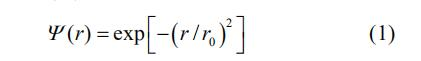Where r0 is the mode field radius of a single-mode fiber; r is the radial distance from any point on the fiber end face to the center. When the light wave transmitted through the long-distance vacuum reaches the receiving aperture, it can be regarded as a plane light wave. The electromagnetic field distribution at the focal point of the receiving lens is: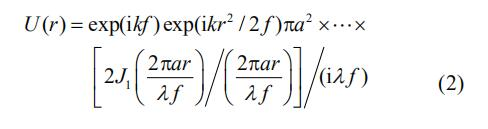Where f is the focal length of the coupling lens; λ is the wavelength; 2a is the aperture stop of the coupling lens; k is the wave number.

The most important condition for efficient coupling of single-mode fiber is to meet the injection conditions (or mode field matching)-the laser coupled into the single-mode fiber has the same electromagnetic field distribution (amplitude and phase) as the single-mode fiber, and can obtain the highest Coupling efficiency. A feasible method is to compare the focal point Airy spot with the mode surface of the fiber end face, and choose appropriate lens parameters to minimize the difference between the airy spot diffracted light field and the mode face of the fiber end face.

The spatial light-single mode fiber coupling efficiency is: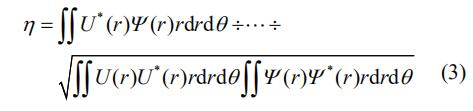Substituting equations (1) and (2) into equation (3), the space light-single-mode fiber energy coupling efficiency is: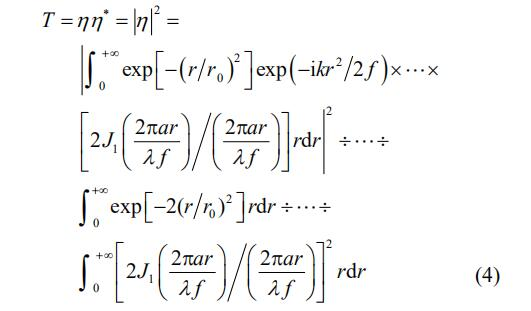From equation (4), it can be seen that when single-mode fiber and light wavelength are selected, the energy coupling efficiency can be changed by changing the value of 2a / f (that is, the inverse of the relative aperture). Due to the complexity of analytic expressions, the search for the highest coupling efficiency is performed by computer numerical calculations. Through calculation, a relationship curve between 2a / f and energy coupling efficiency T is made, as shown in Figure 1.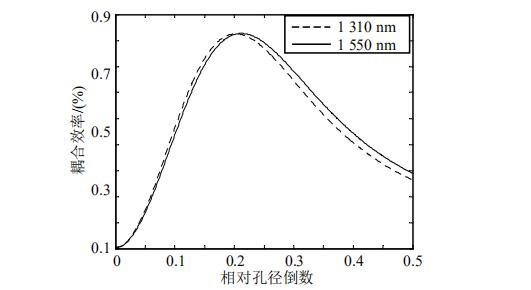Fig. 1 Curve of the relationship between the spatially-single-mode fiber alignment efficiency and the inverse of the coupling lens relative aperture

From Figure 1, read out at 1310 nm and 1550 nm wavelengths, the maximum coupling efficiency and the corresponding reciprocal (optimized value) of the corresponding coupling lens is shown in Table 1.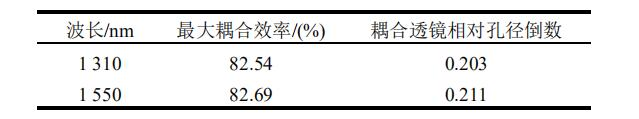Table 1 Reciprocal of the relative aperture of the coupling lens corresponding to the maximum coupling efficiency of single-mode fiber

### Author